# Adjacency list representation of graph

## Adjacency List Representation

Suppose a graph is sparse, then an adjacency list is the better solution for graph representation. In adjacency list representation, for each vertex, we maintain a list of all adjacent vertices. If the edges have weights, then this extra information is also stored in the list cells. Memory space required for adjacency list is O(|E|+|V|) where E represent the number of edges and V represent the number of vertices.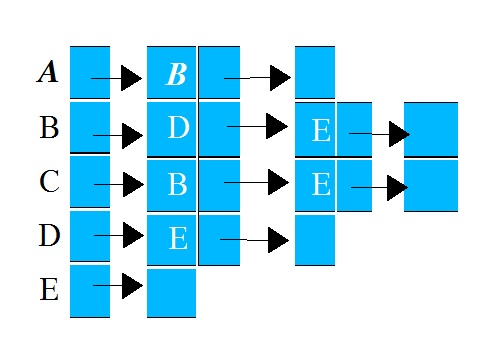Adjacency List Representation of Directed Graph

# Adjacency list representation of graph

## Adjacency List Representation

Suppose a graph is sparse, then an adjacency list is the better solution for graph representation. In adjacency list representation, for each vertex, we maintain a list of all adjacent vertices. If the edges have weights, then this extra information is also stored in the list cells. Memory space required for adjacency list is O(|E|+|V|) where E represent the number of edges and V represent the number of vertices.Adjacency List Representation of Directed Graph

### << Previous Next >>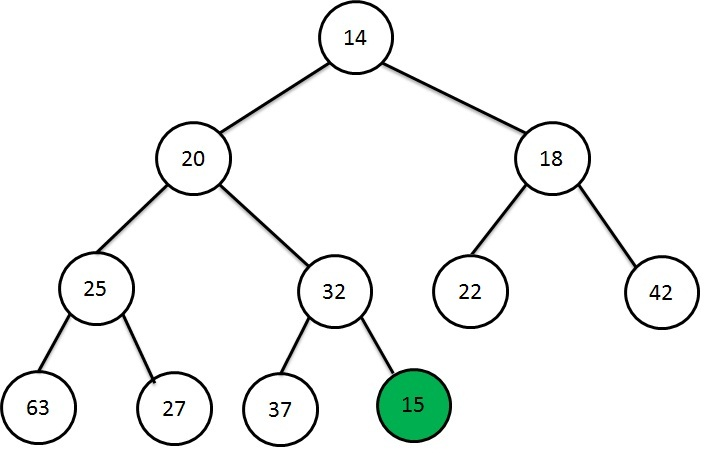Heap Data Structure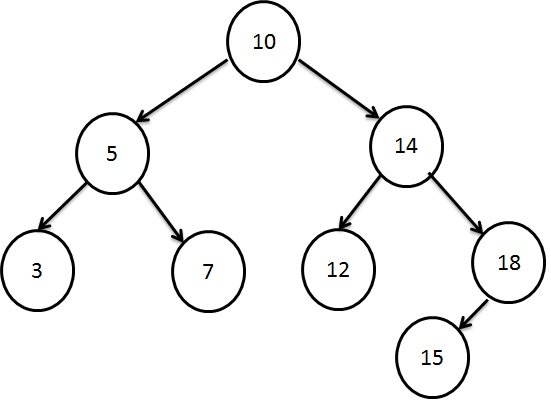Searching using Binary Search Tree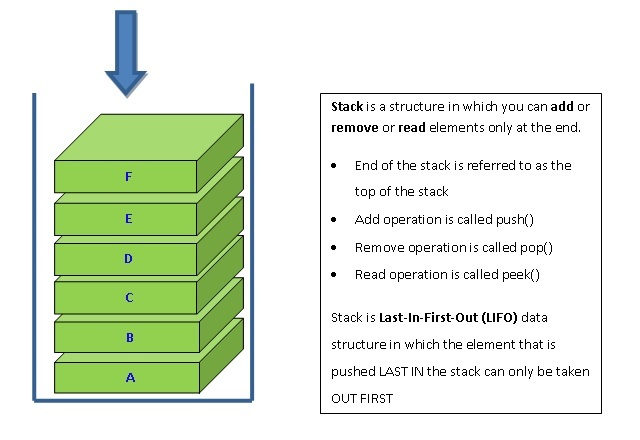Stacking the Data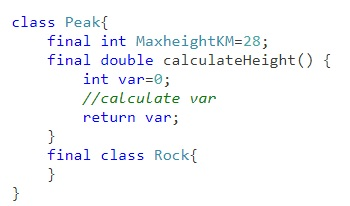Learn Java final keyword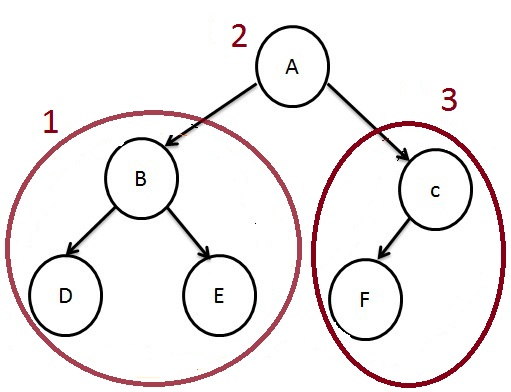How to traverse a Tree?